Compare 2 Columns Through VBA in Microsoft Excel

In case you are wondering how to compare 2 columns of data having rows in millions & extract the unique values among both the columns then you should read this article. We will use VBA code to compare two columns of data & show the difference in next two columns.

Question: There are multiple values which exist in columns A & B. I want a macro to check more than 40 k rows of data in both of the columns & then extract the list of unique entries from each of the column into the next column i.e. columns C & D respectively. In this example, I am taking only 40 rows of sample data.

You can find original question here

Following is the snapshot of data: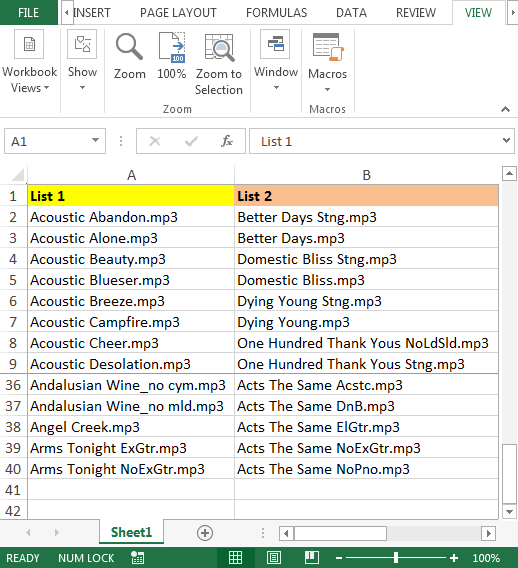To compare the list of two columns, we need to follow the below steps to launch VB editor:

• Click on Developer tab
• From Code group select Visual Basic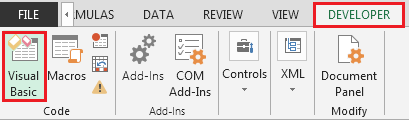• Copy the below code in the standard module

```Sub PullUniques()
Dim rngCell As Range
For Each rngCell In Range("A2:A40")
If WorksheetFunction.CountIf(Range("B2:B40"), rngCell) = 0 Then
Range("C" & Rows.Count).End(xlUp).Offset(1) = rngCell
End If
Next
For Each rngCell In Range("B2:B40")
If WorksheetFunction.CountIf(Range("A2:A40"), rngCell) = 0 Then
Range("D" & Rows.Count).End(xlUp).Offset(1) = rngCell
End If
Next
End Sub
```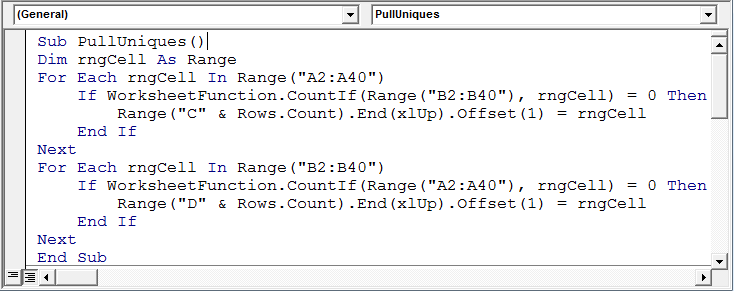• The above macro is all set to run; either we can press F5 key –if you are currently in Visual Basic Editor Screen –or we can use shortcut keys “ALT + F8”, select your macro & click on run button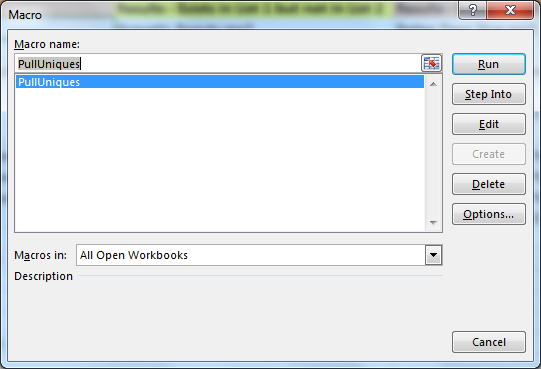• The unique list from columns A & B will be generated automatically in columns C & D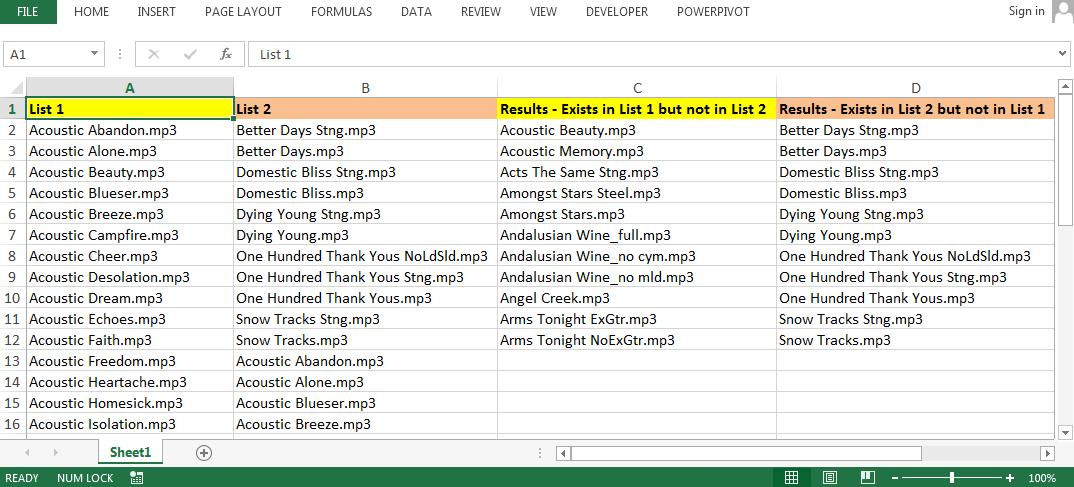Code Explanation:

In the above code, we will use IF function along with For loop to get the result.

• We declare Dim rngCell As Range; the purpose of declaring rngCell As Range is we want to assign the sample range “A2:A40” with a specific name i.e. “rngCell” in our example
• For Each rngCell In Range(“A2:A40″); we will check for each cell in “rngCell” with a condition
• If WorksheetFunction.CountIf(Range(“B2:B40″), rngCell) = 0 Then; this line of code is using COUNTIF VBA function to check criteria range B2:B40 with criteria rngCell is equal to zero, then in column C, the value stored in rngCell will be saved
• Similarly, we will run For Each Loop for column B & extract the unique values in column D

Conclusion: We can get the unique list of text or values from each of the column; the header name of the column C (Results – Exists in List 1 but not in List 2) & column D (Results – Exists in List 2 but not in List 1). In case we have similar requirement but the number of columns are more than two then we need to tweak the code in order to get the result.

We would love to hear from you, do let us know how we can improve, complement or innovate our work and make it better for you. Write us at info@exceltip.com

## Users are saying about us...

1.